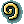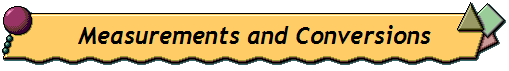Quark: 1 cup = 200g3/4 cup = 150g2/3 cup = 133g½ cup = 100g1/3 cup = 67g¼ cup = 50g Sugar: 1 cup = 200g3/4 cup = 150g2/3 cup = 133g½ cup = 100g1/3 cup = 67g¼ cup = 50g Flour: 1 cup = 125g3/4 cup = 94g2/3 cup = 83g½ cup = 63g1/3 cup = 42g¼ cup = 32g Butter: 1 stick = 113g7 Tbsp = 98g6 Tbsp = 84g5 Tbsp = 70g1/2 stick = 4 Tbsp = 56g3 Tbsp = 42g2 Tbsp = 28g1 Tbsp = 14gOther ingredients: Bread crumbs, 1 cup = 108gChopped nuts, 1 cup = 145gCornstarch, 1 cup = 128gCornstarch, 1 Tbsp = 8gCream of Wheat, 1 cup = 180gGelatine, 1 pack Knox = 17 sheetsRaisins, 1 cup = 165gRice, uncooked, 1 cup = 195g Fluids: 4 cups = 1000ml = 1l2 cups = 500m = 1/2l1 cup = 250ml = 1/4l¾ cup = 188ml2/3 cup = 167ml½ cup = 125ml = 1/8l1/3 cup = 83ml¼ cup = 63ml Temperature: 148C = 300F163C = 325F176C = 350F190C = 375F204C = 400F218C = 425F232C = 450F 1 pack Dr. Oetker Vanilla sugar = 1 ½ tsp home made vanilla sugar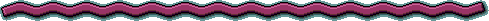[What is Quark?] [Nutritional Info] [How to Make It] [FAQ] [Measurements] [Base Recipes] [Quark Recipes] [Dr. Budwig] [Other Diets] [Resources]German Corner LLC, P.O. Box 70, Keno, OR 97627, USA  Phone: 541-850-0304 copyright © German Corner LLC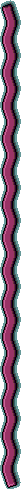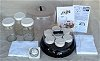Quark Maker Deluxe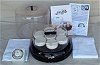Quark Maker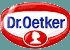German Food Ingredients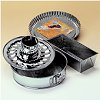German Cooking Utensils